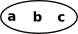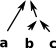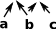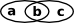# Lattice

This is the algebration of poset. A big difference between
this lattice domain and the poset domain is that, in this domain, the
REP holds a single node whereas in poset REP holds the whole poset.

### Finite and Infinite Lattices

I would like to implement both finite and Infinite lattices:

• Finite lattices are implemented by specifying a poset in the type constructor of the lattice.
• Infinite lattices are implemented by having a recursively defined representation to allow hierarchical expressions to be built up.

Use Bounded versions of Lattice if it has a 'top'/bottom which will be the case for all finite lattices but not infinite lattices since, for finite, we can do a meet/join of all values.

#### Examples

For example consider the integers implemented by:

• zero() -> %
• increment(%) -> %
• decrement(%) -> %

This constructs a partial order. In this order both meet and join are guaranteed to exist (glb and lub) for any finite set of elements. However top and bottom don't exist (∞ and -∞ are not integers). in other words we can't take a meet or a join of an infinite set of elements.

Another example might be a 'tree', this has a bottom element (root) and joins (including infinite) exist. but meets do not exist and the top element does not exist.

The general case of these examples are recursively defined types.

The following intuitionistic logic code is a sort of 'free' lattice.

#### Implementation of Intuitionistic Logic

This is infinite in the sense that it is a sort of 'free' lattice.

In OpenAxiom Gaby implemented PropositionalFormula using Kernel like this:

```PropositionalFormula(T: SetCategory): Public == Private where
....
Rep == Union(T, Kernel %)```

I was considering implementing this as discussed on this thread but, if I understand Waldeks reply correctly, there would be little benefit in doing so and it would involve extra complexity of using sparsely documented code like Kernel.

### Example of the Use of a Frame in Computer Science

See page here for an example of a frame taken from Vickers section 3.7

### Topology

The requirements for the existence of of meets and joins correspond to the requirements for the existence of unions and intersections of open sets. Therefore these lattice structures can represent topologies.

Lattice Open Setinvalid - every intersection should be an open set:### Subset Lattice

This lattice domain implements a subset structure (powerset). The poset equivalent would be a 'containment order'.

One reason for implementing this domain is to investigate the (pseudo)complement.

Here is an test session to show how it works:

 ``` -- first setup some variables SL := SubsetLattice(NNI,[1,2,3])``` ``` (1) SubsetLattice(NonNegativeInteger,[1,2,3]) Type: Type a := subsetLattice()\$SL``` ``` (2)  Type: SubsetLattice(NonNegativeInteger,[1,2,3]) b := subsetLattice()\$SL``` ``` (3)  Type: SubsetLattice(NonNegativeInteger,[1,2,3]) -- test meet and join a/\b``` ``` (4) [1,2] Type: SubsetLattice(NonNegativeInteger,[1,2,3]) a\/b``` ``` (5) [] Type: SubsetLattice(NonNegativeInteger,[1,2,3]) -- test complement complement(a)``` ``` (6) [2,3] Type: SubsetLattice(NonNegativeInteger,[1,2,3]) complement(a)\/complement(b)``` ``` (7)  Type: SubsetLattice(NonNegativeInteger,[1,2,3]) ```

### Infinite (unbounded) Lattices

#### Arbitrary vs. Finite Conjunction and Disjunctions

This structure makes a distinction between arbitrary and finite conjunction/disjunctions.
This is related to the convergence of a sequence (Hausdorff Umgebungsaxiome).

For example: a sequence of ever-smaller open intervals around zero: (-1,1), (-1/2,1/2), (-1/3,1/3), ...

For unions:

• If we take any union of any of these open sets we will get an open set (any point in that union will have a little neighbourhood around it).

For intersections:

• If we take a finite number of the sets and take their intersection, then we'll still get an open set.
• If we take the infinite intersection we don't necessarily get an open set, in the example above the only point that is in all the intervals is 0. 0 on its own isn't an open set, because 0 doesn't have a little neighbourhood around it.

We cannot determine if arbitrary (infinite) frames are equal because, however many terms of two frames that start with the same values we have, they might be still be extended with different values. We can never check the complete frame because we cannot hold an infinite structure in a finite computer.

#### Algebra of Frames

Translating this into lattice algebra:
If A and B are semi-decidable then:

• Conjunction A/\B: if A and B are semi-decidable, then A/\B is semi-decidable (you can test A/\B by testing A and B separately and stopping if and when both the tests for A and B stop).
• Conjunction arbitrary arity /\(Ai): infinite conjunction are not semi-decidable. Note: that even given that we can arbitrarily many tests at the same time, it still doesn't’t follow that arbitrary conjunctions of semi-decidable propositions are semi-decidable: if the first one terminates after 1 minute, the second after 2, etc., we’ll never be able to stop the test of the conjunction even though all tests terminate eventually.
• Disjunction A\/B: if A and B are semi-decidable, then A\/B is semi-decidable
• Disjunction arbitrary arity \/(Ai): is semi-decidable. Given Ai, we can test \/(Ai) by running all the tests in parallel and stopping when any of them stop.
• Implication: A->B isn’t necessarily semi-decidable for the same reason that ¬A isn’t, but we still want to reason about the case where A implies B. Therefore, we allow A->B with the meaning that A implies B, but only at the 'top level' (you can’t nest this connective).
• Top is semi-decidable.
• Bottom is semi-decidable.
• Not: this algebra does not have 'not'/'complement' because if A is semi-decidable, it’s not necessarily the case that ¬A is.

See 

#### Distributive Lattices

Not every lattice is distributive but those that are have some interesting properties which can be investigated by using these domains.

If the lattice is distributive then we can take advantage of this and make the representation more efficient by representing it as either:

meet-of-joins

(a\/b\/...) /\ (c\/d\/...) /\ ...

join-of-meets

(a/\b/\...) \/ (c/\d/\...) \/ ...

So the representation is a list of lists like this:

```Rep := List List Union(_
const : Record(val : Symbol), _
var : Record(str : String) _
)
```

For a finite lattice then:
if meet is distributive over join, then join is also distributive over meet.
and
if join is also distributive over meet, then meet is distributive over join.

We can therefore always convert between join-of-meets and meet-of-joins by 'multiplying' out the terms and applying the idempotence + absorption axioms.

We therefore only need one of these domains to represent a distributive lattice structure. However I have provided both to allow conversions between the two types to be provided.

I look at this like a polynomial for lattices and these conversions are like solving a polynomial.

Here is an test session to show how it works:

 ```-- first setup some variables MOJ := LatticeMeetOfJoins (1) LatticeMeetOfJoins Type: Type a := variable("a")\$MOJ (2) ("a") Type: LatticeMeetOfJoins b := variable("b")\$MOJ (3) ("b") Type: LatticeMeetOfJoins c := variable("c")\$MOJ (4) ("c") Type: LatticeMeetOfJoins d := variable("d")\$MOJ (5) ("d") Type: LatticeMeetOfJoins -- test 'and' land := a /\ b (6) ("a")/\("b") Type: LatticeMeetOfJoins -- opposite landOp := land::LatticeJoinOfMeets (7) ("a"/\"b") Type: LatticeJoinOfMeets -- test or lor := a \/ b (8) ("a"\/"b") Type: LatticeMeetOfJoins -- opposite lorOp := lor::LatticeJoinOfMeets (9) ("a")\/("b") Type: LatticeJoinOfMeets -- test idempotence (should return 'a') a /\ a (10) ("a") Type: LatticeMeetOfJoins a \/ a (11) ("a") Type: LatticeMeetOfJoins -- test absorption (should return 'a') a/\(a\/b) (12) ("a") Type: LatticeMeetOfJoins a\/(a/\b) (13) ("a") Type: LatticeMeetOfJoins -- test composite contructions landor := land \/ c (14) ("a"\/"c")/\("b"\/"c") Type: LatticeMeetOfJoins landorOp := landor::LatticeJoinOfMeets (15) ("a"/\"b")\/("c") Type: LatticeJoinOfMeets landorOpOp := landorOp::LatticeMeetOfJoins (16) ("a"\/"c")/\("b"\/"c") Type: LatticeMeetOfJoins landorand := landor /\ d (17) ("a"\/"c")/\("b"\/"c")/\("d") Type: LatticeMeetOfJoins landorandOp := landorand::LatticeJoinOfMeets (18) ("a"/\"b"/\"d")\/("c"/\"d") Type: LatticeJoinOfMeets landorandOpOp := landorandOp::LatticeMeetOfJoins (19) ("a"\/"c")/\("b"\/"c")/\("d") Type: LatticeMeetOfJoins ```